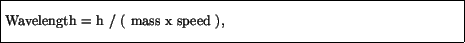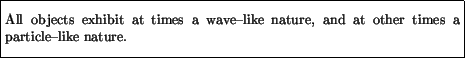Next: Electrons as particles Up: Quantum Theory Previous: Stimulated emission

# Wave particle duality

The Bohr model of the atom involved two puzzling features - the electron was treated as a wave, and light was treated as a particle (a photon). The connection to these new pictures of electrons and light from our more familiar view of an electron as a particle and light as a wave is provided by the relationwhich links the mass and speed of an electron to the wavelength of the associated wave, and by the relationwhich links the frequency of a light wave to the energy of the associated photon.

These relations are not derivable from other relations; they are hypothesized, and are true'' only so long as they satisfy experimental verification. Even so, they are unlike most mathematical statements, however, in the following sense. A statement like Newton's 2nd law of motion,refers on both sides of the equal sign to the same object: one says the force on some object is equal to the mass of the object times the acceleration of the object. Implicit in this is the fact that both sides of the equation are referring to the same object, and so we have in mind one common picture of the object. However, the preceding quantum relations are referring to different pictures of an object: on one side of the equation we view the object as a particle, and use words to describe the particle's mass, speed, and energy, and on the other side of the equation we view the object as a wave, and use words like wavelength and frequency to describe that wave.

Questions then arise. What is an electron? Is it a particle or a wave? And what is light? A wave or a photon? The answer to these is found in the statement of wave-particle duality:Thus, objects (light, electrons, bowling balls, ...) can at times appear to us as waves, and at other times as particles. In this sense they are neither particles nor waves, in an absolute sense, but only exhibit wave or particle properties, depending on the experiment being performed.

This philosophically challenging statement, which is at the heart of quantum theory, is still actively being debated and researched today. We give now some examples of this dual nature of reality for electrons and for light.Next: Electrons as particles Up: Quantum Theory Previous: Stimulated emission
modtech@theory.uwinnipeg.ca
1999-09-29